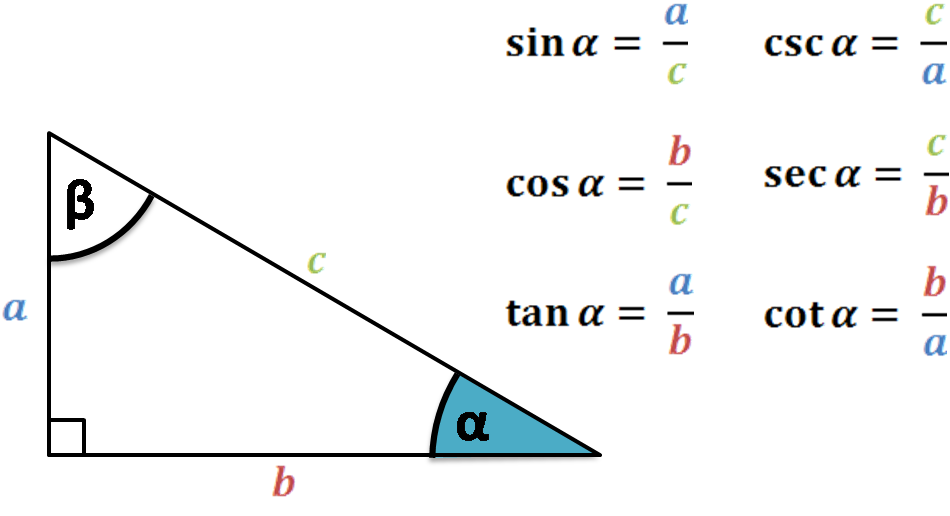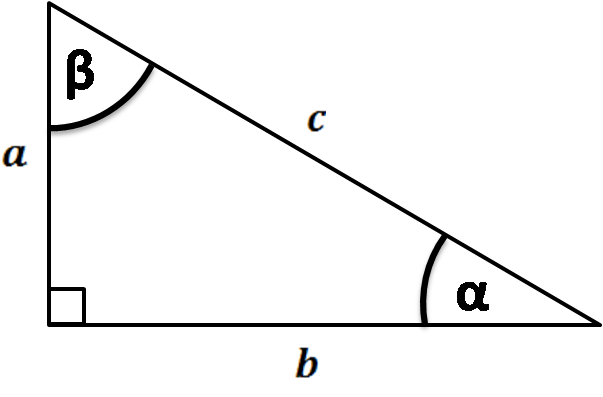# Trigonometry Calculator

Created by Hanna Pamuła, PhD candidate
Reviewed by Bogna Szyk and Jack Bowater
Last updated: Nov 30, 2021

This trigonometry calculator will help you in two popular cases when trigonometry is needed. If you want to find the values of sine, cosine, tangent and their reciprocal functions, use the first part of the calculator. Searching for the missing side or angle in a right triangle, using trigonometry? Our tool is also a safe bet! Type 2-3 given values in the second part of the calculator and in a blink of an eye you'll find the answer. Scroll down if you want to read more about what is trigonometry and where you can apply it.

There are many other tools useful when dealing with trigonometry problems. Check out two popular trigonometric laws: law of sines and law of cosines calculators, helping to solve any kind of a triangle. If you want to read more about the trigonometric functions, go to our dedicated tools:

## What is trigonometry?

Trigonometry is a branch of mathematics. The word itself comes from the Greek trigōnon (which means "triangle") and metron ("measure"). As the name suggests, trigonometry deals mostly with angles and triangles; in particular, it's defining and using the relationships and ratios between angles and sides in triangles. The primary application is thus solving triangles, specifically right triangles, but also any other type of triangle you like.

🔎 Trigonometric functions (sin, cos, tan) are all ratios. Therefore, you can find the missing terms using nothing else than our ratio calculator!

Trigonometry has plenty of applications: from everyday life problems such as calculating the height or distance between objects to the satellite navigation system, astronomy, and geography. Also, sine and cosine functions are fundamental for describing periodic phenomena - thanks to them, we can describe oscillatory movements (as simple pendulum) and waves like sound, vibration or light.Many different fields of science and engineering use trigonometry and trigonometric functions, to mention only a few of them: music, acoustics, electronics, medicine and medical imaging, biology, chemistry, meteorology, electrical, mechanical and civil engineering, even economics... The trigonometric functions are really all around us!

## Trig calculator finding sin, cos, tan, cot, sec, csc

To find the trigonometric functions of an angle, enter the chosen angle in degrees or radians. Underneath the calculator, six most popular trig functions will appear - three basic ones: sine, cosine and tangent, and their reciprocals: cosecant, secant and cotangent. Additionally, if the angle is acute, the right triangle will be displayed, which can help you in understanding how the functions may be interpreted.

## Trigonometry calculator as a tool for solving right triangle

To find the missing sides or angles of the right triangle, all you need to do is enter the known variables into the trigonometry calculator. You need only two given values in the case of:

• one side and one angle
• two sides
• area and one side

Remember that if you know two angles, it's not enough to find the sides of the triangle. Two triangles having the same shape (which means they have equal angles) may be of different sizes (not the same side length) - that kind of relationship is called triangle similarity. If the sides have the same length, then the triangles are congruent.

## FAQ

### What is trigonometry?

Trigonometry is the study of the relationships within a triangle. For right angled triangles, the ratio between any two sides is always the same, and are given as the trigonometry ratios, cos, sin, and tan. Trigonometry can also help find some missing triangular information, e.g., the sine rule.

### How to do trigonometry?

1. Find which two out of hypotenuse, adjacent, opposite and angle you have.
2. Work out which of the remaining options you are trying to calculate.
3. Choose which relationship you need (remember, SOHCAHTOA).
4. Fill in the data you have into the equation.
5. Rearrange and solve for the unknown.

### Is trigonometry hard?

Trigonometry can be hard at first, but after some practise you will master it! Here are some trigonometry tips: label the hypotenuse, adjacent and opposite on your triangle to help you figure out what identity to use, and remember the mnemonic SOHCAHTOA for the trigonometric relationships!

### What is trigonometry used for?

Trigonometry is used to find information about all triangles, and right angled triangles in particular. Since triangles are everywhere in nature, trigonometry is used outside of math, in fields such as construction, physics, chemistry engineering and astronomy.

### Who invented trigonometry?

Since trigonometry is the relationship between angles and sides of a triangle, no one invented it, it would still be there even if no one knew about it! The first people to discover part of trigonometry were the Ancient Egyptians and Babylonians, but Euclid and Archemides first proved the identities, although they did it using shapes, not algebra.

Trigonometry is usually taught to teenagers aged 13-15, which is grades 8 & 9 in the USA and years 9 & 10 in the UK. The exact age at which trigonometry is taught depends on the country, school, and ability of the pupils.

### How to convert decimal to degrees in trigonometry?

1. Find which trigonometric relationship you are using with SOHCAHTOA.
2. Take the inverse identity of you decimal, e.g., sin-1(0.5).
3. The resulting number is the degree of your angle.
4. Check your results with Omni Calculator.

### How to find the height of a triangle using trigonometry?

1. Draw your triangle and mark the height. You will have split the triangle into two smaller triangles.
2. Solve either of these remaining triangles using regular trigonometry to find the height. Either the opposite or adjacent will now be the hypotenuse of the smaller triangle.
Hanna Pamuła, PhD candidate
Trigonometric functions: sin, cos, tan...
Angle
deg
...or trigonometry in right triangle
Given
two sides
a
in
b
in
c
in
Angle α
deg
Angle β
degPeople also viewed…

### Meat footprint

Check out the meat impact - on the environment and your health.

### Secretary problem (Valentine's day)

Use the dating theory calculator to enhance your chances of picking the best lifetime partner.

### Slant height of a cone

With our slant height of a cone calculator finding the measurements of a cone has never been easier!

### Trapezoid perimeter

Find the perimeter of a trapezoid with any of the possible combination of inputs with our trapezoid perimeter calculator!